# Business Intelligence Visualizations with Python

Go to the source link to view the article. You can view the picture content, updated content and better typesetting reading experience. If the link is broken, please click the button below to view the snapshot at that time.

# Business Intelligence Visualizations with Python

## I prepared an extensive guide to display beautiful visualizations to enhance metrics, KPIs, forecasts, and other insights.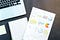Photo by Lukas from Pexels

Why are visualizations worth thousands of words? They have the power of telling stories and simplifying the interpretation of information. They help users detect patterns, insights and metrics, and as a result build better products and services. So they do really matter.

Visualizations are one of the most powerful tools in the set available to data analysts or data enthusiasts. In order to facilitate their creation, a wide range of softwares and languages has been developed. Maybe the usefulness of visualizations relates to the different interpretation that our brain naturally gives to images instead of large DataFrames, arrays or traditional tables allotted with data.

Importance of visualizations. (2 min read)

Introduction to plot types with Python (8 min read)

# 1. Importance of Visualizations

Tabulated data complicates conclusion extraction. Isolated numbers from their contexts, although structured in columns and rows accordingly to provide structure and orientate the user, are hard to make meaning out of. On the other hand, visualizations represent values at a glance. They show tabulated data in a simple way to easily and rapidly compare values, facilitating decision making.

More important are these skills in Finance, Econometrics, Data Analytics and other math-related fields in which decision making is based on numeric fundamentals, usually hard to explain to not a savvy finance team member.

Imagine yourself as an Asset Manager in an Investment Committee explaining your data-driven approach to asset allocation that creates an optimized portfolio with Machine Learning algorithms. Surely visualizations come in handy as argumentation for studies.

Big-data and huge-volume data processing have leveled-up the bar when it comes to storytelling-complexity of conclusions extracted from the analysis. In this context, user-friendly reports and other tailored presentations that can be recreated to specific audiences gain special value.

In this article I will not focus on specifically-designed tools to produce visualizations such as Tableau, Qlik and Power BI. Products within this category vary by capabilities and ease–of–use and are generally quick to set up, enabling users to access data from multiple sources. I will majorly focus in bringing you insights to more tailor-made visualizations with the application of our coding skills in Python, taking advantage of the Matplotlib a 2-D plotting library which has some neat tools to enable the creation of beautiful and flexible graphs and visualizations.

Let’s take a look on how data-interpretation is hugely improved with the use of data. Have a look at this DataFrame including different types of tea’s sales:

In the following image you’ll see how it would be visually displayed with a Matplotlib bar-plot. Clearly, rapid conclusions can be made, such as best and worst selling tea-types, intermediate-selling ones and a comparisons between the magnitude of the sales of each tea type at a glance: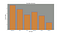# 2. Introduction to plot types with Python

Installation process is pretty straight forward. Just open your terminal and insert the following command:

`pip install matplotlib`

## A. Line Plot

After having installed the library, we can jump on to plot creation. The first type we’re going to create is a simple Line Plot:

`# Begin by importing the necessary libraries:import matplotlib.pyplot as plt`

Suppose you want to plot your company’s one week expenditure compared to the previous week’s one the with the following input data:

`# Days of the week:days = [1, 2, 3, 4, 5, 6,7]# Money spend one week 1money_spent = [1000, 1200, 1500, 1080, 1400, 1650, 1350]# Money spend one week 2money_spent_2 = [900, 1500, 1200, 1050, 950, 1250, 1000]# Create figure:fig = plt.figure(figsize=(10,5))# Plot first week expenses:plt.plot(days, money_spent)# Plot second week expenses:plt.plot(days, money_spent_2)# Display the result:plt.title('Company Expenditure Comparison')plt.legend(['First week', 'Second week'])plt.show()`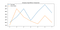Sample plot — Image by Author

This plot can have some nice styling modifications with few lines of code:

`# Display the result:ax = plt.subplot()ax.set_xticks(range(1,8))ax.set_xticklabels(['Monday', 'Tuesday', 'Wednesday', 'Thursday', 'Friday','Saturday',' Sunday'])plt.title('Company Expenditure Comparison')plt.legend(['First week', 'Second week'])plt.show()`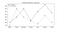If you want to zoom-in to a particular spot you can do it with the plt.axis command with the input of the X and Y axis desired coordinates:

`plt.axis([1,3,900,1600])`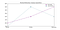## Subplots

Matplotlib library provides a way to plot multiple plots on a single figure. In order to create multiple plots inside one figure, configure the following arguments for the matplotlib.pyplot.subplots method as a generalization:

`# Subplot creationplt.subplots(nrows=1,ncols=1,sharex=False,sharey=False,squeeze=True,subplot_kw=None,gridspec_kw=None)`

In case you don’t insert these parameters as they’re set by default, be sure to utilize the plt.subplot method to indicate the coordinates of the subplot to configure. Parameters:

• nrows: Number of rows in the figure.
• ncols: Number of columns in the figure.
• plot_number: Index of the subplot inside the figure.

For e.g., suppose that you want to make a representation of temperature across the year in relation to flight sales during the same period with the following input data, just to find out if there’s any correlation between those variables:

`# Temperature and flight sales months = range(12)temperature = [37, 38, 40, 53, 62, 71, 78, 74, 69, 56, 47, 48]flights = [1100, 1300, 1200, 1400, 800, 700, 450, 500, 450, 900, 950, 1100]# Create figure:fig = plt.figure(figsize=(12,6))# Display the result - Plot 1:plt.subplot(1,2,1)# Plot temperatures:plt.plot(months,temperature,color='steelblue',linestyle='--')# Configure labels and title:plt.xlabel('Months')plt.ylabel('Temperature')plt.title('Temperature Representation')# Display the result - Plot 2:plt.subplot(1,2,2)# Plot flights:plt.plot(months,flights,color='red',marker='o')plt.xlabel('Month')# Configure labels and title:plt.ylabel('Flights Summary')plt.title('Flights per month')plt.show()`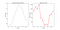Sample plot — Image by Author

## B. Side-by-side Bar Chart

I’ll skip visualizations of simple bar charts to focus on more business-related side-by-side charts. The basic command utilized for bar charts is plt.bar(x_values, y_values).

Side-by-side bar charts are used to compare two sets of data with the same types of axis values. Some examples of data that side-by-side bars could be useful for include:

• Population of more than one country over a period of time.
• Prices for different foods at more than one restaurant over a period of time.
• Enrollments in different classes for male and female students.

We will use the following information to create the chart:

`# Values for X axis bar separation:x_values1 = [0.8,2.8,4.8,6.8,8.8,10.8]x_values2 = [1.6,3.6,5.6,7.6,9.6,11.6]# Sales by month and labels:drinks = ["cappuccino", "latte", "chai", "americano", "mocha", "espresso"]months_sales = ['Jan','Mar','May','Jun','Aug','Oct', 'Dec']sales_cappuccino = [95, 72, 53, 62, 51, 25]sales_latte = [62, 81, 34, 62, 35, 42]`

Plot configuration looks something like this:

`# Figure creation:fig = plt.figure(figsize=(12,8))# Subplot configuration:ax = plt.subplot()ax.set_xticks(range(1,12,2))ax.set_xticklabels(months_sales)# Bar plot creation:plt.bar(x_values1, sales_cappuccino, color='gray')plt.bar(x_values2, sales_latte, color='purple')# Display plot:plt.title("Coffee Sales Comparison")plt.xlabel("Types of coffees")plt.ylabel("Pounds sold")plt.legend(labels=drinks, loc='upper right')plt.show()`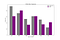## C. Stacked Bars Chart

If we want to compare two sets of data while preserving knowledge of the total between them, we can also stack the bars instead of putting them side by side. We do this by using the keyword bottom.

For e.g. suppose that we want to evaluate the distribution of our sales by product without losing perspective from total sales:

`# Product identification & Sales per product:product = ['Computer', 'Keyboard', 'Headset', 'Mouse', 'Monitor']sales_c = np.random.randint(1000,3000,5)sales_k = np.random.randint(1000,3000,5)sales_h = np.random.randint(1000,3000,5)sales_m = np.random.randint(1000,3000,5)sales_o = np.random.randint(1000,3000,5)# Configure bottoms to stack bars:k_bottom = np.add(sales_c, sales_k)h_bottom = np.add(k_bottom, sales_h)m_bottom = np.add(h_bottom, sales_m)# Create figure and axes:fig = plt.figure(figsize=(10,8))ax = plt.subplot()# Plot bars individually:plt.bar(range(len(sales_c)),sales_c, color='#D50071', label=product)plt.bar(range(len(sales_k)),sales_k, bottom=sales_c, color='#0040FF',label=product)plt.bar(range(len(sales_h)),sales_h, bottom=k_bottom, color='#00CA70',label=product)plt.bar(range(len(sales_m)),sales_m, bottom=h_bottom, color='#C14200',label=product)plt.bar(range(len(sales_o)),sales_o, bottom=m_bottom, color='#F0C300',label=product)# Display graphs:ax.set_xticks(range(5))ax.set_xticklabels(['Monday','Tuesday', 'Wednesday', 'Thursday', 'Friday'])plt.legend(loc='best')plt.title('Sales Distribution by Product')plt.ylabel("Products Sold")plt.show()`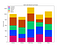## D. Pie Chart

If we want to display elements of a data set as proportions of a whole, we can use a pie chart. In Matplotlib, you can make a pie chart with the command plt.pie, passing in the values you want to chart.

We also want to be able to understand what each slice of the pie represents. To do this, we can either:

• Use a legend to label each color.
• Put labels on the chart itself.

One other useful labeling tool for pie charts is adding the percentage of the total that each slice occupies. Matplotlib can add this automatically with the keyword autopct. In addition, I’ll add the explode feature, which highlights selected pieces of the “pie”.

I’ll use the following data to plot the chart:

`# Sales and regions:region = ['LATAM', 'North America','Europe','Asia','Africa']sales = [3500,5500,4800,4500,2500]# Create figure and plot pie:fig = plt.figure(figsize=(10,8))plt.pie(sales, labels=region,autopct='%d%%', colors=colors,explode=explode_values)plt.axis('equal')plt.title('Global Sales Distribution', fontsize='20')plt.savefig('plot_eight.png')plt.show()`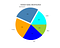## E. Histogram

A histogram tells us how many values in a dataset fall between different sets of numbers, for example how many numbers fall between 0 and 10? This question represents a bin which might be between 0 and 10.

All bins in a histogram are always the same size:

• The width of each bin is the distance between the minimum and maximum values of each bin.
• Each bin is represented by a different rectangle whose height is the number of elements from the dataset that fall within that bin.

The command plt.hist finds the minimum and the maximum values in your dataset and creates 10 equally-spaced bins between those values by default. If we want more than 10 bins, we can use the keyword bins to set the instruction.

A problem we face is that our histograms might have different numbers of samples, making one much bigger than the other. To solve this, we can normalize our histograms using normed=True.

In the example below, I include a credit scoring case in which we want to visualize how scores are distributed among both groups of clients. I tailored the histogram to create 12 bins instead of the default 10 and to set alpha or transparency level at 0.5 in order to see both histograms at the same time avoiding overlapping distributions.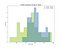## F. Stacked Plot

This type of chart plots the variables of a table or timetable in a stacked plot, up to a maximum of 25 variables. The function plots the variables in separate y-axes, stacked vertically. The variables share a common x-axis.

I’m going to simulate the case of the evolution of market share for three companies for the last eight years, with the respective visualization in the stacked plot:

`# Insert DataFrames:year = pd.DataFrame(range(2013,2021),columns=['Year'])volume1 = pd.DataFrame([1000,1100,1200,1250,1300,1350,1400,1450], columns=['Values 1'])volume2 = pd.DataFrame([1000,900,800,700,600,500,400,300], columns=['Values 2'])volume3 = pd.DataFrame([1000,950,900,850,800,750,700,650], columns=['Values 3'])# Create main frame:frames = [year,volume1, volume2, volume3]frame = pd.concat(frames,axis=1)# Plot axis, labels, colors:x_values = frame['Year']y_values = np.vstack([frame['Values 1'], frame['Values 2'], frame['Values 3']])labels = ['Company A', 'Company B', 'Company C']colors = ['skyblue', 'peru', 'gray']# Display plot:fig = plt.figure(figsize=(10,8))plt.stackplot(x_values, y_values, labels=labels, colors=colors ,edgecolor='black')plt.title('Market Share Evolution',fontsize=15)plt.ylabel('Share', fontsize=15)plt.legend(loc='best')plt.show()`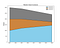## G. Percentage Stacked plot

Also known as a 100% Stacked Area Chart, this chart displays the trend of the percentage each value contributes over time or categories.

In this case, we’ll plot the same information as in the Stacked Plot but with the percentage contributed to the total market share by each company: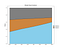# Conclusion

With the posted guidelines and plot types in this article, I hope to have helped you to enhance your data analytics skills, at least from a data-visualization stand point.

This is the beginning of a series of articles related to data-visualization that I’ll be preparing to share more insights and knowledge. If you liked the information included in this article don’t hesitate to contact me to share your thoughts. It motivates me to keep on sharing!

# Reference

Thanks for taking the time to read my article! Any question, suggestion or comment, feel free to contact me: [email protected]